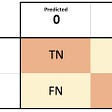# The technique of Gradient Descent

1. Learning rate — The rate at which the descent on the graph occurs, higher learning rate can imply faster descent on the graph which tends to miss the local minima during the gradient descent
2. Epoch — The number of times you wish to revise the hyperparameters in order to reach the best possible hyperparameter
1. Theta new — The new value of theta which is obtained after the iteration of gradient descent which yields more accurate predictions
2. Theta old — The old value of theta which has to be updated
3. Negative sign — This always guides the algorithm in the right direction, because if the derivative of the cost function is positive, then the gradient descent algorithm will direct the theta towards the left which points in the direction of decreasing cost whereas if the derivative of the cost function is negative, the algorithm will direct the theta towards the right which points in the direction of decreasing cost
4. Learning rate — This term determines the size of the steps taken in the direction of the minima to find the parameter with the least cost
5. Derivative — This term determines the magnitude of the differentiation which also plays a role in determining the steps taken in the direction of the minima to find the parameter with the least cost
1. Linear Regression
1. Theta 0 is the intercept
2. Theta1 is the parameter/coefficients of regression
3. X is the input
1. m is the number of training examples
2. h(theta) is the hypothesis function
3. y is the target variable
• Positive and negative errors might cancel out each other, hence taking the square of the errors prevents the errors from being cancelled
• The errors less than 1 in magnitude are reduced and the errors greater than 1 in magnitude are increased imposing more penalty for those errors and hence helping our model to learn in a better way

--

--

--

## More from Aayushmaan Jain

A data science enthusiast currently doing bachelor's degree in data science

Love podcasts or audiobooks? Learn on the go with our new app.

## [PR 113] GHVAE: Greedy Hierarchical Variational Autoencoders for Large-Scale Video Prediction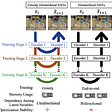## How to use the Support Vector Machine (SVM) as a classifier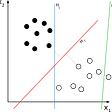## Artificial Intelligence can be used to track the progression of diabetes## Sentiment Analysis on IMDb reviews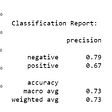## Which Political Party Has AI Predicted Will Win The 2024 Presidential Race?## Identify the Dog’s breed using Neural Network## An Introduction to Text Summarization using the TextRank Algorithm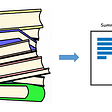## From Keras to PyTorch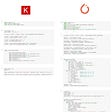## Aayushmaan Jain

A data science enthusiast currently doing bachelor's degree in data science

## A python function to get all the possible stats from a confusion matrix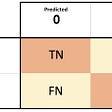## Mistakes In DataVisualization: A Short Practical Review (1)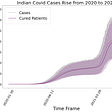## Best Performance Metrics To Evaluate Your Machine Learning Algorithms